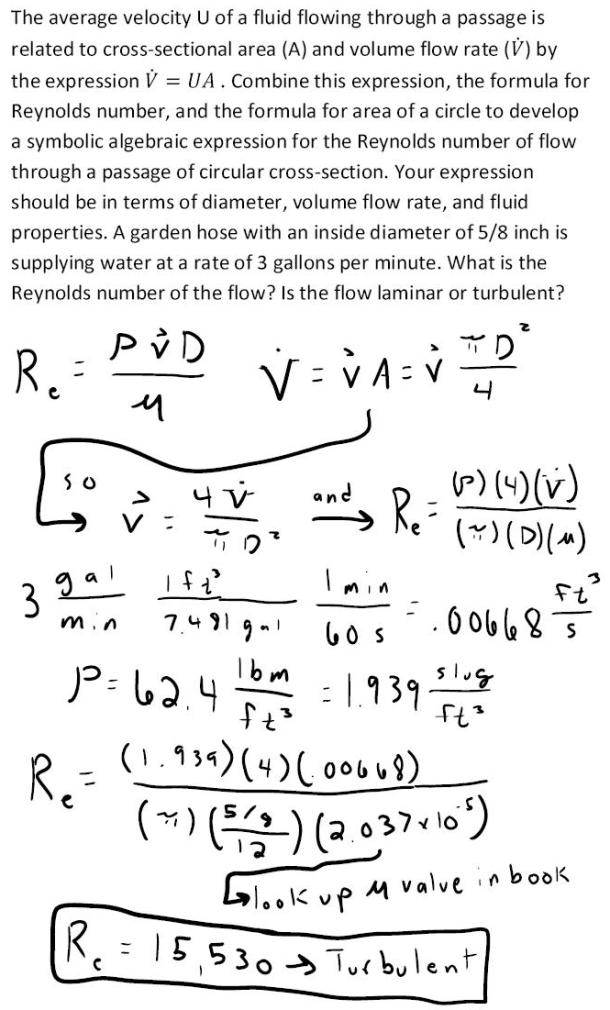fluid mechanics math problems engineering equations fluid mechanics basic mechanics school homework engineering math fluids formulas fluid mechanics problems fluid mechanics problem solutions to fluids problems full solution fluid mechanics engineering fluids problem solution fluid mechanics math problems engineering equations fluid mechanics basic mechanics school homework engineering math fluid mechanics formulas fluid problems fluid mechanics problem solutions to fluid mechanics problems full solution fluid mechanics engineering fluid mechanics problem solution
fluid mechanics math problems engineering equations fluid mechanics basic mechanics school homework engineering math fluids formulas fluid mechanics problems fluid mechanics problem solutions to fluids problems full solution fluid mechanics engineering fluids problem solution fluid mechanics math problems engineering equations fluid mechanics basic mechanics school homework engineering math fluid mechanics formulas fluid problems fluid mechanics problem solutions to fluid mechanics problems full solution fluid mechanics engineering fluid mechanics problem solution
Highalphabet Home Page fluid mechanics solutions fluids math solved Fluid Mechanics Page 1
The average velocity U of a fluid flowing through a passage is related to cross-sectional area (A) and volume flow rate (V dot) by the expression V dot=UA. Combine this expression, the formula for Reynolds number, and the formula for area of a circle to develop a symbolic algebraic expression for the Reynolds number of flow through a passage of cirrcular cross-section. Your expression should be in terms of diameter, volume flowrate, and fluid properties. A garden hose with an inside diameter of 5/8 inch is supplying water at a rate of 3 gallons per minute. What is the Reynolds number of the flow? Is the flow laminar or turbulent?The average velocity U of a fluid flowing through a passage is related to cross-sectional area (A) and volume flow rate (V dot) by the expression V dot=UA. Combine this expression, the formula for Reynolds number, and the formula for area of a circle to develop a symbolic algebraic expression for the Reynolds number of flow through a passage of cirrcular cross-section. Your expression should be in terms of diameter, volume flowrate, and fluid properties. A garden hose with an inside diameter of 5/8 inch is supplying water at a rate of 3 gallons per minute. What is the Reynolds number of the flow? Is the flow laminar or turbulent?